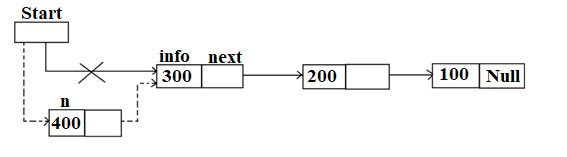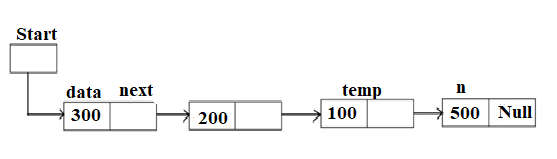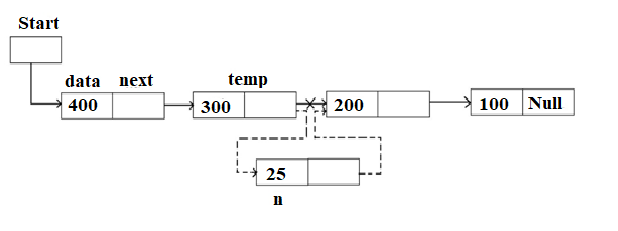The following basic operations can be performed on linked list:

2. Insert an element in the linked list
3. Delete an element from the linked list
5. Searching an element in the linked list

#### Operations on Linear singly Linked list

Inserting an element in the list:

There are three positions where an element can be inserted. Following figures explains the inserting an element in the list at beginning, at the end and at given position.

1. Insert at the beginningn->next=start;

start=n;

1. Insert at endn->next=NULL;

temp->next=n;

1. Insert at given positionn->next=temp->next;

temp->next=n;

#### //Program: Following program creates linear singly linked list and displays the same.

#include<stdio.h>

#include<conio.h>

#include<malloc.h>

struct node

{

int info;

struct node *next;

};

typedef struct node NODE;

NODE *start=NULL;

void main()

{

char ch=’Y’;

int data;

NODE *n, *temp;

clrscr();

while(ch==’y’ || ch==’Y’)

{

n=(NODE *)malloc(sizeof(NODE));

printf(“\n Enter the data to store: ”);

scanf(“%d”,&data);

n->next=NULL;

n->info=data;

if(start==NULL)

{

temp=start=n;

}

else

{

temp->next=n;

temp=n;

}

fflush(stdin);

printf(“\n Do you want to continue ? (Y / N): “);

ch=getchar();

}

printf(“\n Linked list is : \n”);

temp=start;

while(temp->next!=NULL)

{

printf(“%d->”,temp->info);

temp=temp->next;

}

printf(“%d”, temp->info);

getch();

}

#### /*Program: Following program shows the operations insert at beginning, at end and in between in linear singly linked list and displays the same.*/

#include<stdio.h>

#include<conio.h>

#include<malloc.h>

struct node

{

int info

struct node *next;

};

typedef struct node node

NODE *start=NULL;

void main()

{

int ch;

void display();

clrscr();

while(1)

{

clrscr();

printf(“\n1. Insert at begining”);

printf(“\n2 Insert at end”);

printf(“\n3. Insert in between”);

printf(“\n4. Display”);

printf(“\n5. Exit”);

scanf(“%d”,&ch);

switch(ch)

{

break;

break;

break;

case 4: display();

break;

case 5: exit(0);

default: printf(“\n Please enter correct choice…”);

break;

}

}

getch();

}

#### // Insert data at beginning

{

int data;

NODE *n;

printf(“\n Enter data to store :”);

scanf(“%d”,&data);

n=(NODE *)malloc(sizeof(NODE));

n->info=data;

if(start==NULL)

{

n->next=NULL;

start=n;

}

else

{

n->next=start;

start=n;

}

getch();

}

// Insert data at end

{

int data;

NODE *n, *temp;

printf (“\n Enter data to store : “);

scanf(“%d”, &data);

n=(NODE *)malloc(sizeof(NODE));

n->next=NULL;

n->info=data;

temp=start;

while(temp->next!=NULL)

temp=temp->next;

temp->next=n;

getch();

}

#### //Insert data in between

{

int i,pos,data;

NODE *n, *temp;

printf(“\n Enter position to insert: “);

scanf(“%d”,&pos);

temp=start;

for(i=1;i<pos-1;i++)

{

temp=temp->next;

if(temp==NULL)

{

printf(“\n There are less than %d elements..”,pos);

}

}

printf(“\n Enter data to store :”);

scanf(“%d”,&data);

n=(NODE *)malloc(sizeof(NODE));

n->next=temp->next;

n->info=data;

temp->next=n;

getch();

}

void display()

{

NODE *temp;

if(start==NULL)

{

}

temp=start;

while(temp!=NULL)

{

printf(“%d->”,temp->info);

temp=temp->next;

}

getch();

}

#### Some More:

POP- Introduction to Programming Using ‘C’

DS – Data structure Using C

OOP – Object Oriented Programming

Java Programming

DBMS – Database Management System

RDBMS – Relational Database Management System